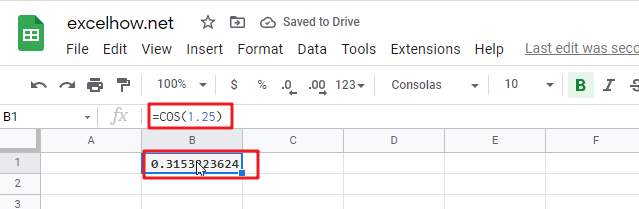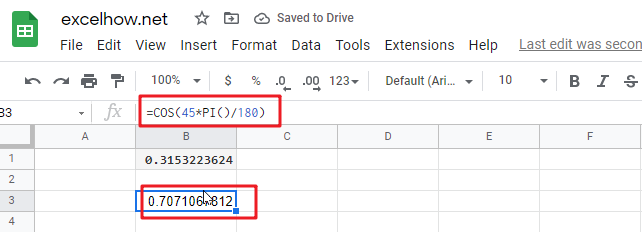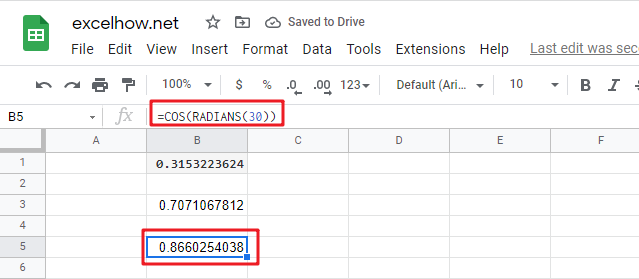# ExcelHow

This post will guide you how to use Google Sheets COS function with syntax and examples.

## Description

The Google Sheets COS function returns the cosine of a given angle. If you want to supply an angle to COS function in degrees, then you need to multiply the angle by PI()/180. Or you can use the RADIANS function to the angle to radians.

The purpose of this function is used to get the cosine of an angle provided in radians in google sheet and it’s returned value is the cosine value.

The COS function is a build-in function in Google Sheets and it is categorized as a MATH function.

## Syntax

The syntax of the COS function is as below:

= COS(number)

Where the COS function argument is:

• numberThis is a required argument. A number that used to be calculate the cosine of an angle.

Note:

• If your argument is in degrees, multiply it by PI()/180 or use the RADIANS function to convert it to radians.

## Google Sheets COS Function Examples

The below examples will show you how to use google sheets COS Function to return the cosine of an angle.

1# get the cosine of 1.25 radians, enter the following formula in Cell B1.

`=COS(1.25)`2# get the cosine of 45 degrees, enter the following formula in Cell B2.

`=COS(45*PI()/180)`3# get the cosine of 30 degrees, enter the following formula in Cell B3.

`=COS(RADIANS(30))`